# Week 4: Electronics Production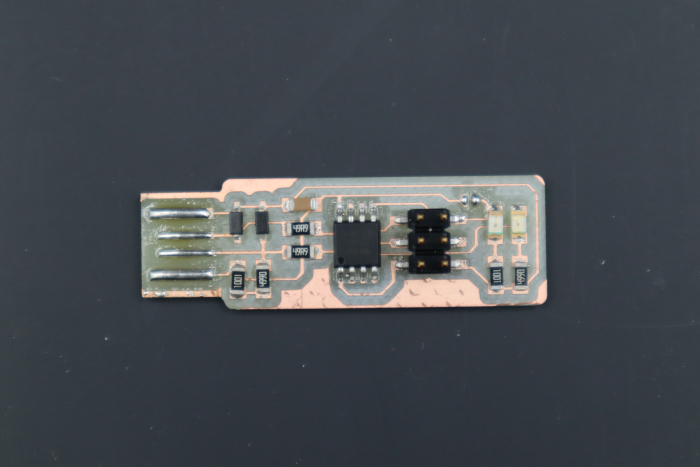Hero Shot

This week we were tasked with milling and sodering a pcb, as well as testing and making sure it could program a another one of its kind if need be. This will help us get experience in milling boards with the Roland and sodering which will be invaluable in the weeks to come.

# Design Rules for PCB Production

Feed Rate: Feed rate is characterized by the distance that is travelled when the spindle rotates one revolution. (speed: 4 mm/s)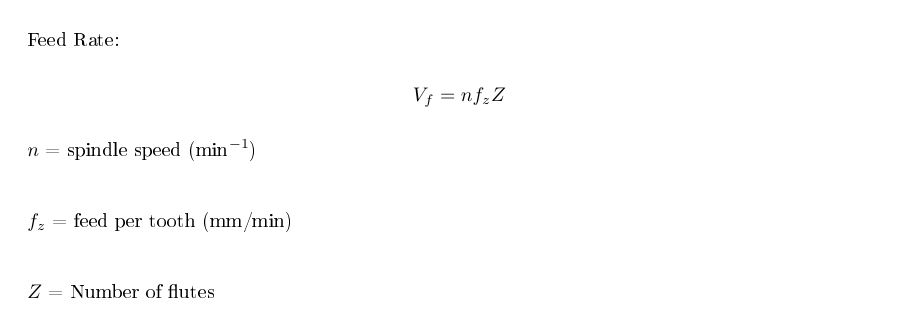Equation for feed rate

Spindle Speed: Spindle speed is how fast the spindle rotates. It is measured in revolutions per minute (RPM)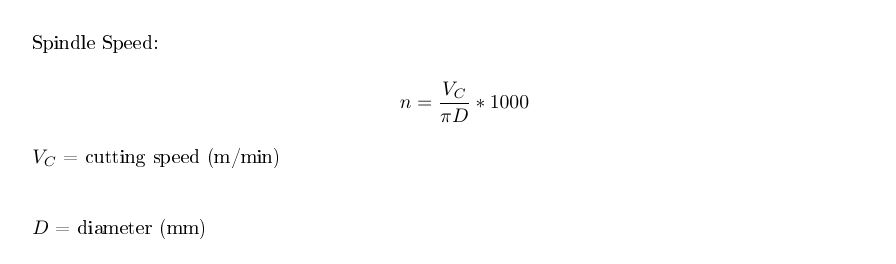Equation for spindal speed

Plunge Rate: The plunge rate is the initial speed that the bit is moved down into the material. The rate is specifically the initial speed since the hardness of the material, the bit, and many other factors can affect the bits movement along the z-axis during the cut.

Depth of Cut: Depth of cut is how deep into a material the end mill cuts each pass. In other words, it’s how much material is removed per pass, and it’s typically measured in mm. If you set the z origin to be the top of the material, the depth of cut is the vertical distance between the origin and the end mill.

• Trace: .004 in
• Outline: .024 in

Tooling: Tooling is the process of designing and manufacturing components necessary to manufacture things down the line (i.e. a mold, jigs, fixtures, etc).

Roland SRM20 Specifications:

• Cutting Material ( + size):
• Roland SRM20 can cut many materials such as PC board, acrylic, poly acetate, ABS, chemical wood, modeling wax, and foam.
• Bed size: 9.14 (X) x 6.17 (Y) inches
• Maximum material height: less than 5.15 in
• Maximum material weight: 4.4 lbs
• Mechanical Resolution: 0.0000393 inches/step
• Software: 0.000039 inches/step (RML-1), 0.000039 inches/step (NC code)
• Operating Speed: 0.24 – 70.87inch/min
• Spindle Rotation Speed: Adjustable 3,000 – 7,000 rpm

More Specifications for our milling machine can be found here

RML Syantax: RML (Roland Machine Language) is a language written by Roland that uses different commands from other cnc languages and was written because Roland was very influential in the beginning of CNC. RML (Mode 1) is written in the following format:

* [command] ** [parameter] ** [,] ** [parameter] ** [terminator]

Where:

• “ * ” is an ASCII character <= “ “
• “ ** “ is either “ “ or “\t” (tab)
• “[,]” is a comma
• “[command]” is a command in the form of a single letter or character and is a command for the Roland Mill
• “[parameter]” is the parameter for the command
• “[terminator]” is formally “CR + LF” and is not necessary when followed by the next command

NC (Numeric Control) Syntax:

% EOB

O[PROGRAM NUMBER] EOB

N[PROGRAM NUMBER] G[PREPARATORY FUNCTION] EOB

N[PROGRAM NUMBER] G[PREPARATORY FUNCTION] X[]Y[]Z[] F[] S[] M[] EOB

N[PROGRAM NUMBER] … … … EOB

… … … EOB

N[PROGRAM NUMBER] M02 EOB

%

Where:

• “%” denotes the start or end of the data
• “O” followed by a series of numbers denotes the program number
• “EOB” indicates the end of a block
• “N” is the sequence number and references the line of the block
• “G” denotes a preparatory function and is followed by a 2-digit number that specifies the function
• “X...Y...Z...” denotes the X, Y, Z value of a coordinate or distance
• “F” denotes the Feed Rate
• “S” denotes the Spindle Speed
• “M” denotes a Miscellaneous Function (rotating, stopping, etc)
• “M02” denotes the end of the program (“M02” or “M30”)

# Desktop Mill Test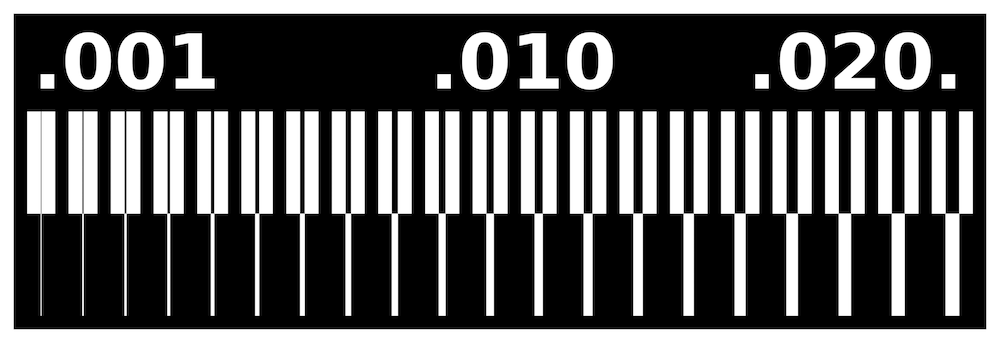Equation for spindal speed

We were tasked with undertaking as test to see exactly how small of lines our desktop mill can make withe the bits that we were given.

Our bits are:

• Traces cut with 1/32" carbide ball nose bit
• speed = 4mm/s
• cut depth = 0.004 in
• Outline cut with 1/32" carbide bit
• speed = 4mm/s
• cut depth = .024 in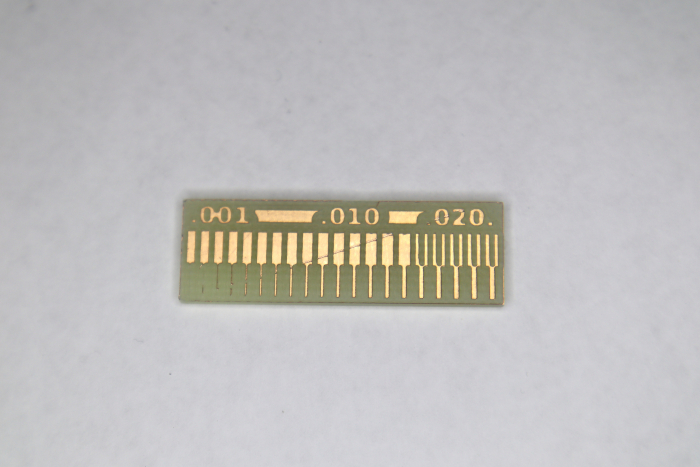Equation for spindal speed

After milling our test, it seems that it could not cut the first four from the left. This is because our bit is too thick and cannot make the precise cuts that were given to it.

# Making my PCB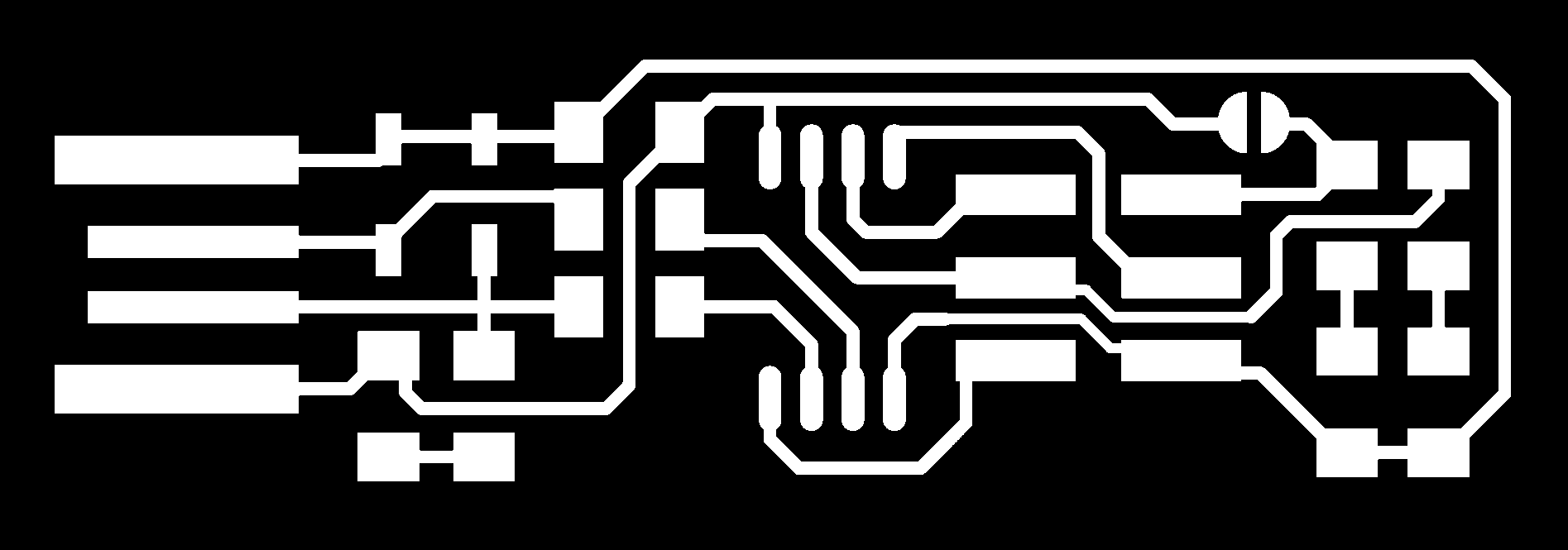Equation for spindal speedEquation for spindal speed

Above are the outline and traces need to cut our the board I want.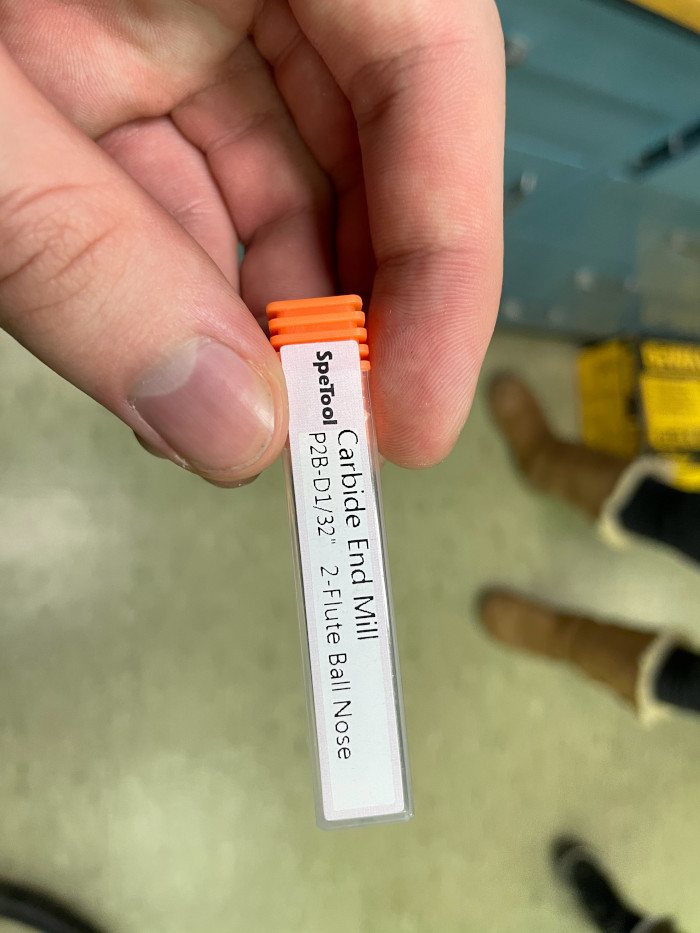Equation for spindal speed

Above is the 1/32" carbide ball nose bit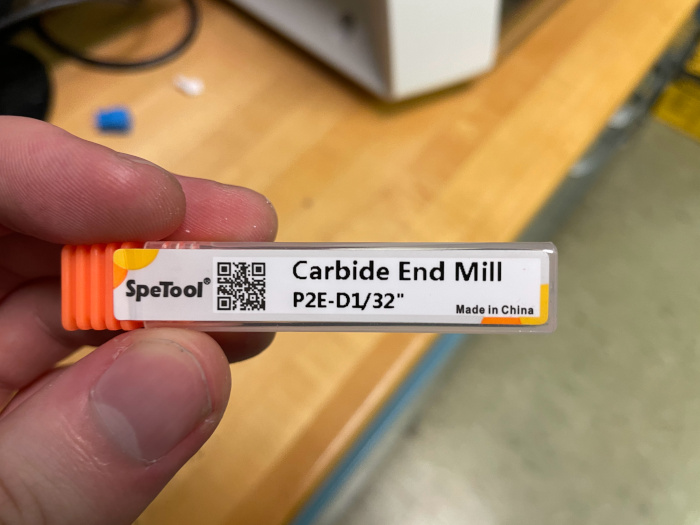Equation for spindal speed

Above is the 1/32" carbide bit

There is a good amount of parts that I need to solder onto my board

Parts I need are

• Two 1k ohm resistors
• Two 499 ohm resistors
• Two 49 ohm resistors
• Two 3.3V Zener Diodes
• 100nF capacitor
• Green LED
• Red LED
• ATtiny45

Other important aspects is that I need to solder over J1, since our zero ohm jumpers are too small at the lab, and I need to solder the USB connections.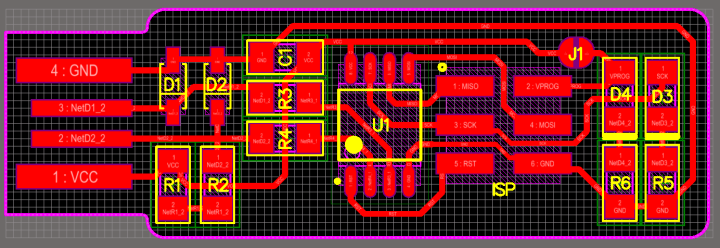Equation for spindal speed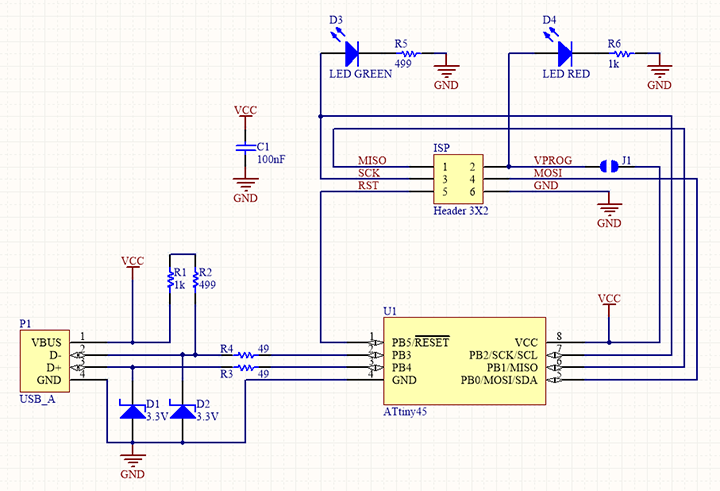Equation for spindal speed

Once I got my pcb milled out it was time to set up my soldering station. Below, you can see that my board came out pretty nice and there was no maintenance I needed to do. I didn't really want to remake the board from scratch, so maintaing a good soldering process was going to be key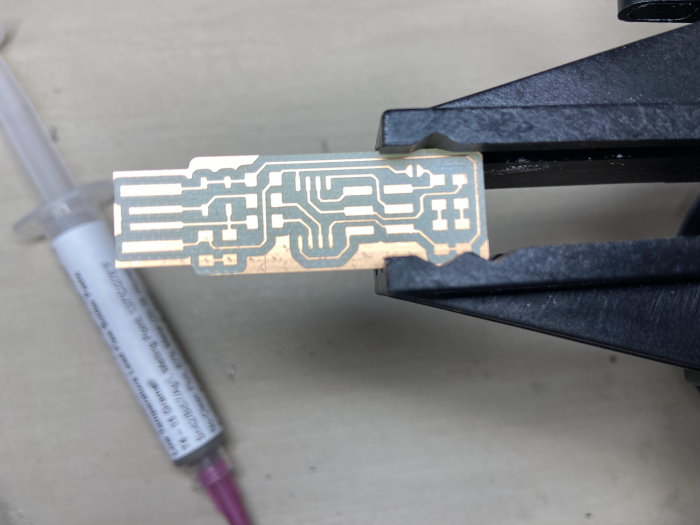Equation for spindal speed

I decided that I wanted to use soldering paste for the majority of my work. Soldering paste ended up being very easy to use once I got the hang of it. At first, I would end up putting too much paste on and my components would end up moving around when I would apply the hot air. This problem was quickly solved when I realized I didn't need much paste at all.

The sticky substance of the solder paste helps to temporarily hold components in place when hot air is applied the paste melts until it turns shiney creating a mechanical bond as well as an electrical connection.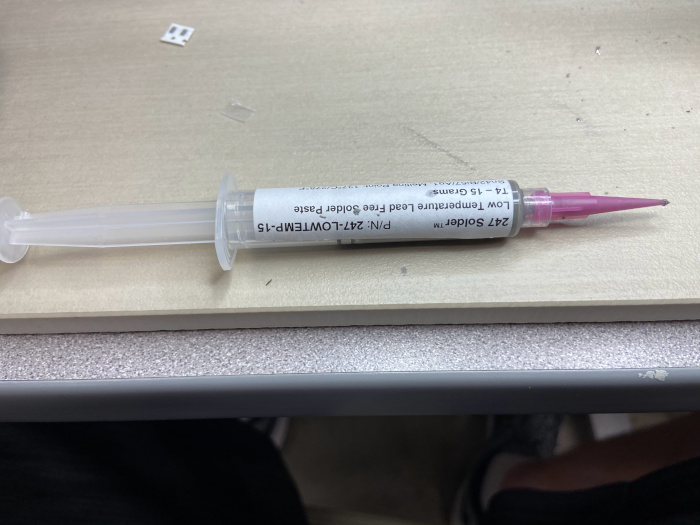Equation for spindal speed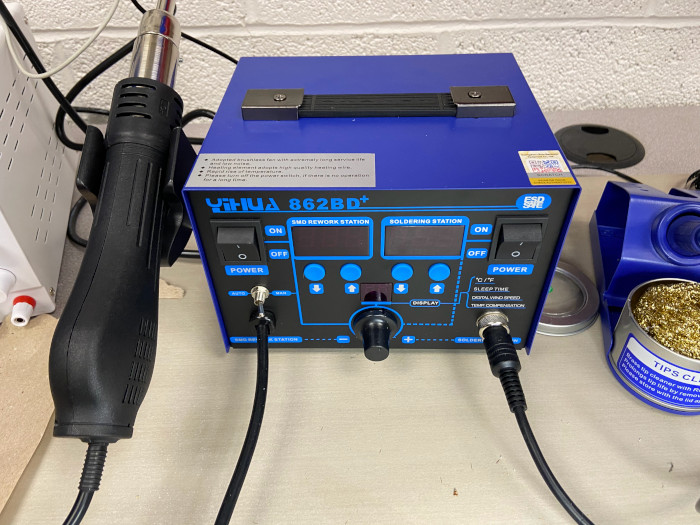Equation for spindal speed

Putting on the first component went pretty well, but I was putting a lot of paste on in the beginning.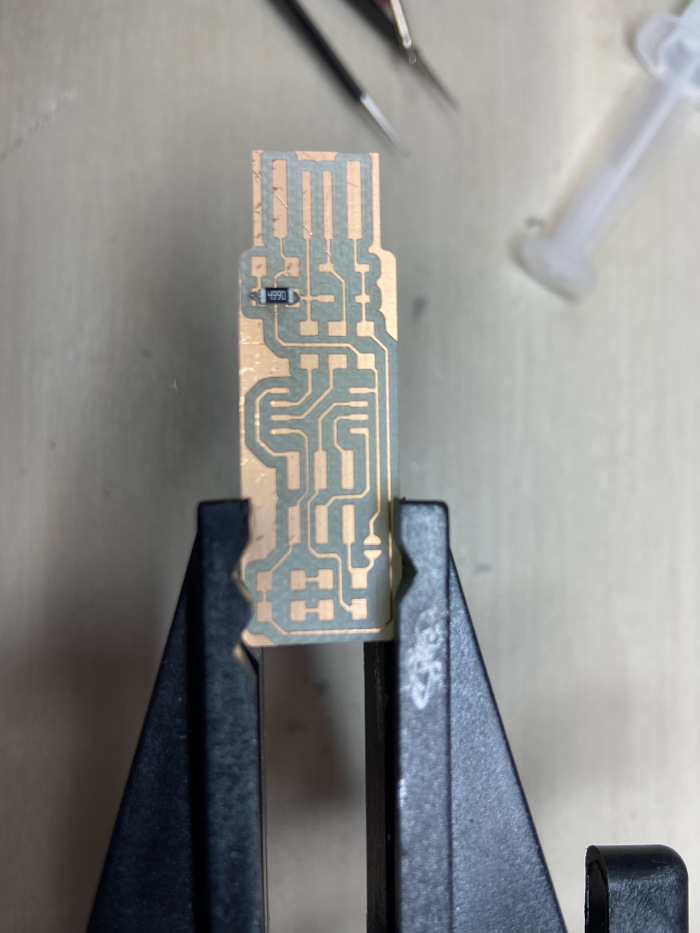Equation for spindal speed

I focused on putting easier components on first even though from what ive been told it is best to work going from the inside out.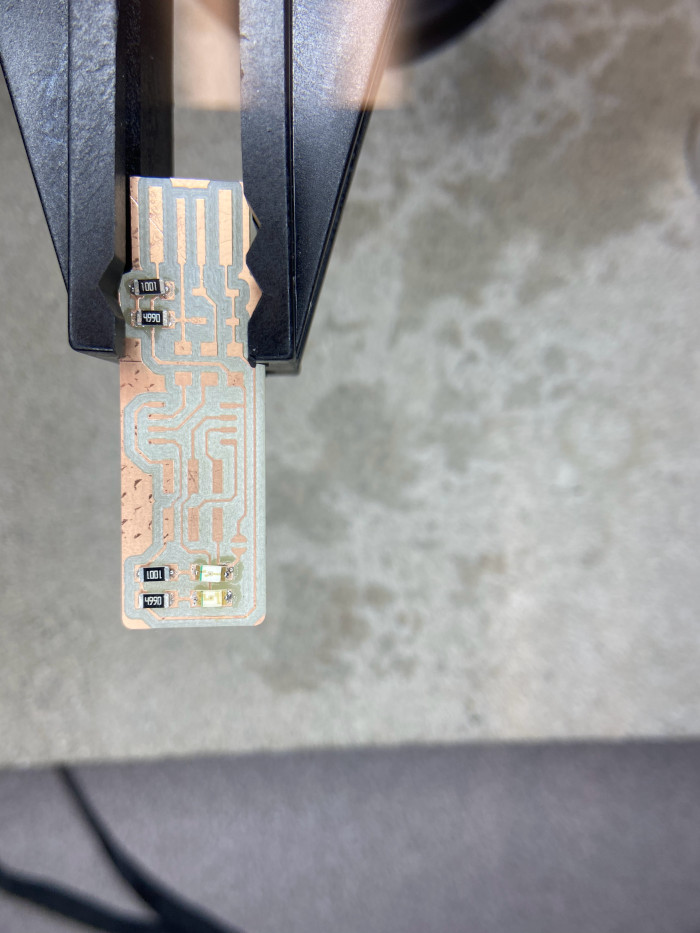Equation for spindal speed

When putting on parts it was imperitive to check the continuity of so we know if we have a complete path for current flow, it also helps to check if you have any unwanted connections. Best way to do this is to put one prong of the multimeter on your component and other prong on the copper you wanted to connect it to. If a electrical connection was made, and your in the continuity setting in the multimeter, you should hear a beep.

below you can see me testing out the LED to make sure they have solid connections.

Once I got he components on I had to make my usb connections. I decided since I had been using soldring paste the entire time, I would use it for the usb connections as well.

I did not have very many problems with putting my components on the board. The only major thing I did wrong was I though the ground would go up for the diodes so I ended putting them on upside down. But it ended up being a pretty quick fix I put hot air to the solder and flipped them around, while making sure I did not pull to early before its melted as you can rip the copper off. I also ended up having continuity between two of the pins on the ATtiny45, but since the solder ball in question wasn't on any copper, I touched it with the iron and it came right off.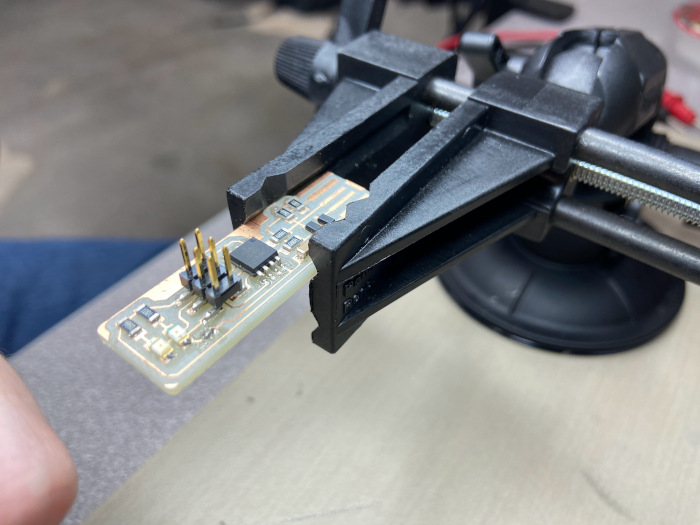Equation for spindal speed

As you can see, I would make a long line of soldering paste along the connection, and when I heated it up, the solder should only want to make conncetions to the copper. A downside to this method is that there was a good amount of flux left over that could affect the longevity of my pcb since it can eat away at the copper.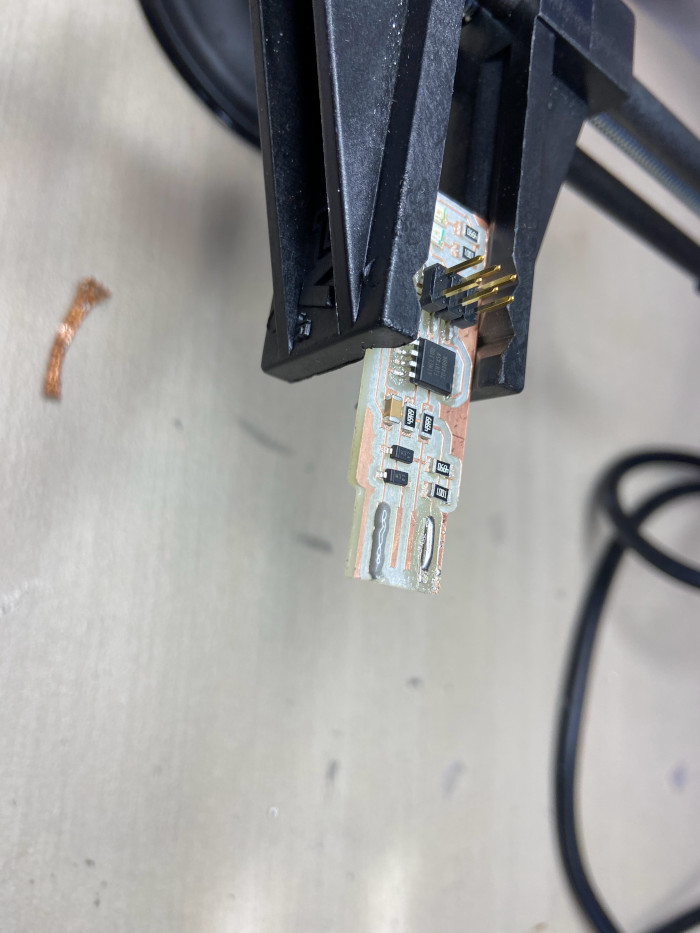Equation for spindal speed

Below is my finished pcb, I now needed to program it.Equation for spindal speed

Plugging it into the computer, I can see that the LED lit up which is good.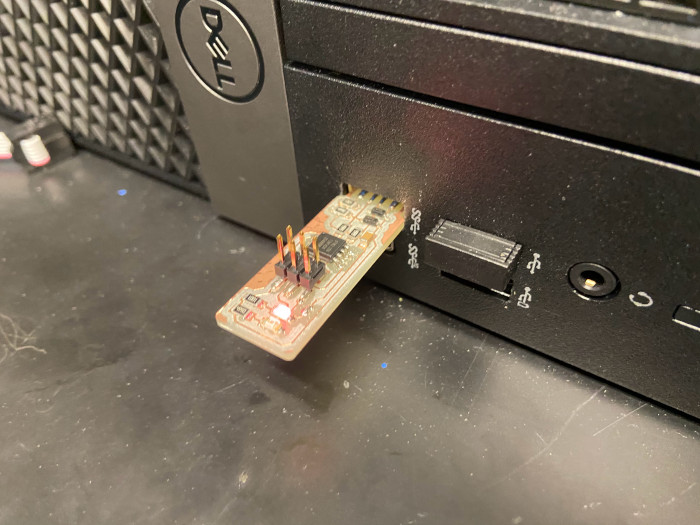Equation for spindal speed

Using the pin connections and an already programmed pcb, I plugged both them into the computer so that I may program my FabTinyISP with one that is already programmed.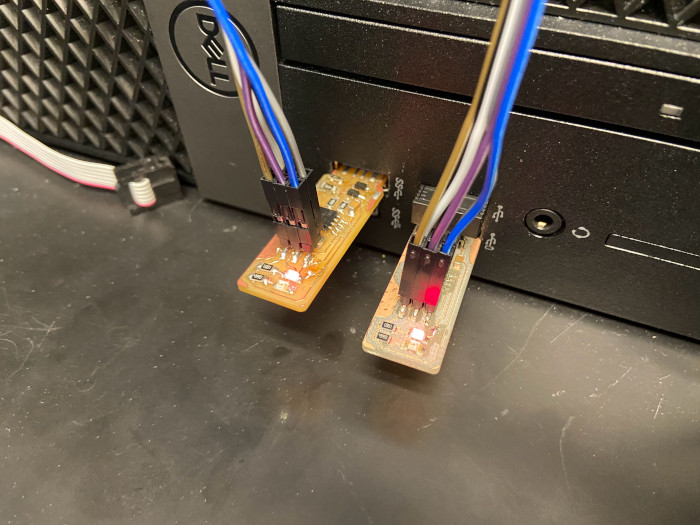Equation for spindal speed

Below is the message I recieved when I blew the first fuse.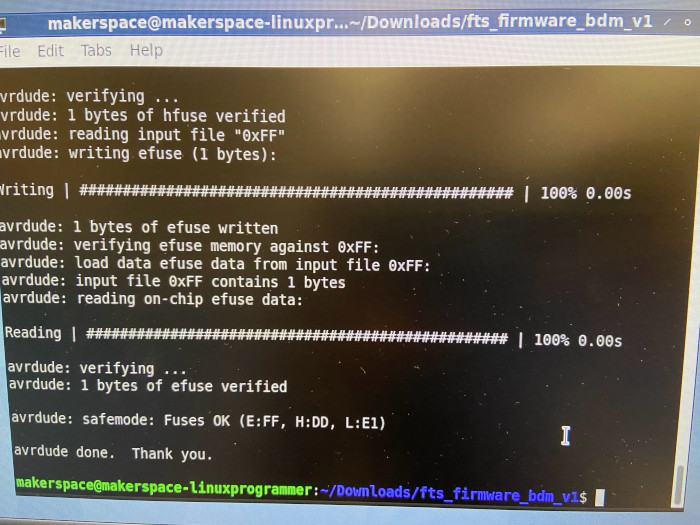Equation for spindal speed

The computer successfully recognizes my devise in the USB port which is good. I also gave my device a serial number (je after my initials) so that I can always differentiate it from others, since we are all making the same thing.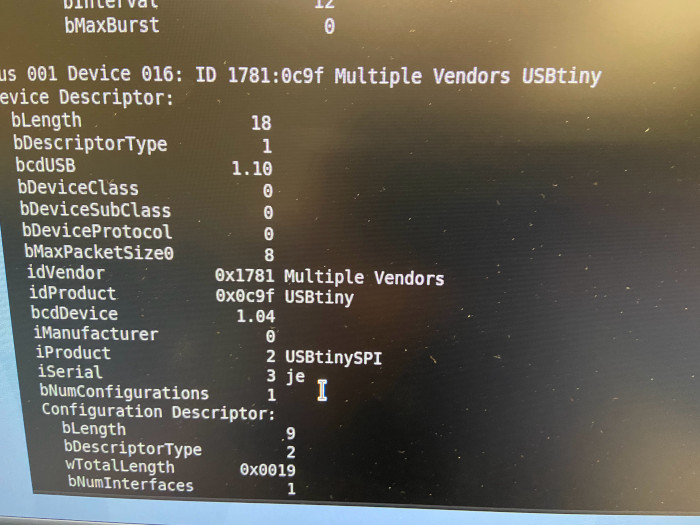Equation for spindal speed

Below I have blown the second fuse thus making it extremley hard for my devise to ever be programmed again. The only remaining step after this is to go back an un-solder the connection I made at J1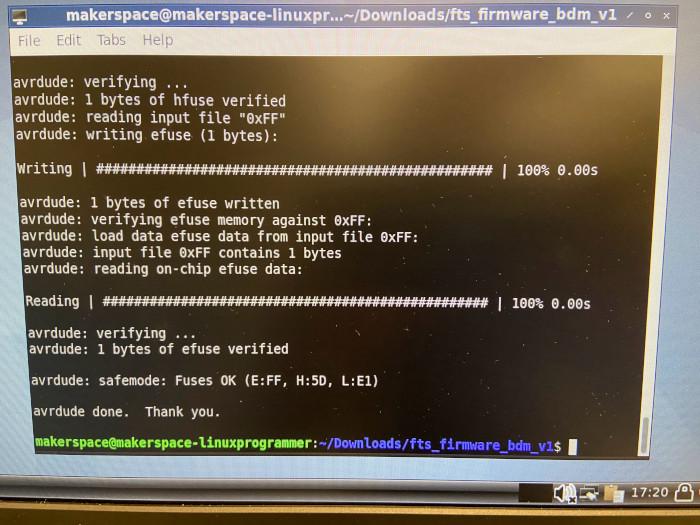Equation for spindal speed

FabTinyISP files

Line test files: